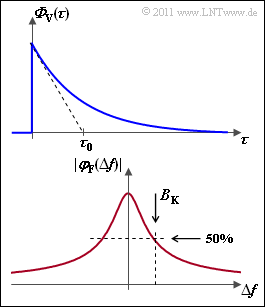# Exercise 2.7: Coherence BandwidthDelay power density spectrum and
frequency correlation function

For the delay power density spectrum, we assume an exponential behavior.  With  ${\it \Phi}_0 = {\it \Phi}_{\rm V}(\tau = 0)$  we have

$${{\it \phi}_{\rm V}(\tau)}/{{\it \phi}_{\rm 0}} = {\rm e}^{ -\tau / \tau_0 } \hspace{0.05cm}.$$

The constant  $\tau_0$  can be determined from the tangent in the point  $\tau = 0$  according to the upper graph.  Note that  ${\it \Phi}_{\rm V}(\tau)$  has unit  $[1/\rm s]$ .  Furthermore,

• The probability density function  $\rm (PDF)$  $f_{\rm V}(\tau)$  has the same form as  ${\it \Phi}_{\rm V}(\tau)$, but is normalized to area  $1$ .
• The  average excess delay or mean excess delay  $m_{\rm V}$  is equal to the linear expectation  $E\big [\tau \big]$  and can be determined from the PDF $f_{\rm V}(\tau)$ .
• The  multipath spread or delay spread  $\sigma_{\rm V}$  gives the standard deviation of the random variable  $\tau$ .  In the theory part we also use the term  $T_{\rm V}$ for this.
• The displayed frequency correlation function  $\varphi_{\rm F}(\Delta f)$  can be calculated as the Fourier transform of the delay power density spectrum  ${\it \Phi}_{\rm V}(\tau)$ :
$$\varphi_{\rm F}(\Delta f) \hspace{0.2cm} {\bullet\!\!-\!\!\!-\!\!\!-\!\!\circ} \hspace{0.2cm} {\it \Phi}_{\rm V}(\tau)\hspace{0.05cm}.$$
• The coherence bandwidth  $B_{\rm K}$  is the value of $\Delta f$ at which the frequency correlation function  $\varphi_{\rm F}(\Delta f)$  has dropped to half in absolute value.

Notes:

$$x(t) = \left\{ \begin{array}{c} {\rm e}^{- \lambda \hspace{0.05cm}\cdot \hspace{0.05cm} t}\\ 0 \end{array} \right.\quad \begin{array}{*{1}c} \hspace{-0.35cm} {\rm for} \hspace{0.15cm} t \ge 0 \\ \hspace{-0.35cm} {\rm for} \hspace{0.15cm} t < 0 \\ \end{array} \hspace{0.4cm} {\circ\!\!-\!\!\!-\!\!\!-\!\!\bullet} \hspace{0.4cm} X(f) = \frac{1}{\lambda + {\rm j} \cdot 2\pi f}\hspace{0.05cm}.$$

### Questionnaire

1

What is the probability density function  $f_{\rm V}(\tau)$  of the delay time?

 $f_{\rm V}(\tau) = {\rm e}^{-\tau/\tau_0}$. $f_{\rm V}(\tau) = 1/\tau_0 \cdot {\rm e}^{-\tau/\tau_0}$, $f_{\rm V}(\tau) = {\it \Phi}_0 \cdot {\rm e}^{-\tau/\tau_0}$.

2

Determine the average delay time for  $\tau_0 = 1 \ \ \rm µ s$.

 $m_{\rm V} \ = \$ $\ \rm µ s$

3

Which value results for the multipath spread with  $\tau_0 = 1 \ \ \rm µ s$?

 $\sigma_{\rm V} \ = \$ $\ \rm µ s$

4

What is the frequency–correlation function  $\varphi_{\rm F}(\Delta f)$?

 $\varphi_{\rm F}(\Delta f) = \big[1/\tau_0 + {\rm j} \ 2 \pi \cdot \delta f \big]^{-1}$, $\varphi_{\rm F}(\Delta f) = {\rm e}^ {-(\tau_0 \hspace{0.05cm}\cdot \hspace{0.05cm}\Delta f)^2}$.

5

Determine the coherence bandwidth  $B_{\rm K}$.

 $B_{\rm K} \ = \$ $\ \ \rm kHz$

### Solution

#### Solution

(1)  Let  ${\it \Phi}_0 = {\it \Phi}_{\rm V}(\tau = 0)$.  The integral of the delay power density spectrum gives

$$\int_{0}^{+\infty} {\it \Phi}_{\rm V}(\tau) \hspace{0.15cm}{\rm d} \tau = {\it \Phi}_{\rm 0} \cdot \int_{0}^{+\infty} {\rm e}^{-\tau / \tau_0} \hspace{0.15cm}{\rm d} \tau = {\it \Phi}_{\rm 0} \cdot \tau_0 \hspace{0.05cm}.$$
• Then the probability density function is
$$f_{\rm V}(\tau) = \frac{{\it \Phi}_{\rm V}(\tau) }{{\it \Phi}_{\rm 0} \cdot \tau_0}= \frac{1}{\tau_0} \cdot {\rm e}^{-\tau / \tau_0} \hspace{0.05cm}.$$
• Solution 2 is correct.

(2)  The  $k$-th moment of an  one-sided exponential random variable  is  $m_k = k! \cdot \tau_0^k$.

• With  $k = 1$, this results in the linear mean value  $m_1 = m_{\rm V}$:
$$m_{\rm V} = \tau_0 \hspace{0.1cm} \underline {= 1\,{\rm µ s}} \hspace{0.05cm}.$$

(3)  According to the  Steiner's Theorem, the variance of any random variable is  $\sigma^2 = m_2 \, -m_1^2$.

• This yields  $m_2 = 2 \cdot \tau_0^2$, and therefore
$$\sigma_{\rm V}^2 = m_2 - m_1^2 = 2 \cdot \tau_0^2 - (\tau_0)^2 \hspace{0.3cm} \Rightarrow \hspace{0.3cm} \sigma_{\rm V} = \tau_0 \hspace{0.1cm} \underline {= 1\,{\rm µ s}} \hspace{0.05cm}.$$

(4)  ${\it \Phi}_{\rm V}(\tau)$  is identical to  $x(t)$  in the given Fourier transform pair if  $t$  is replaced by  $\tau$  and  $\lambda$  by  $1/\tau_0$.

• Thus,  $\varphi_{\rm F}(\Delta f)$  is equal to  $X(f)$  with the substitution  $f → \Delta f$:
$$\varphi_{\rm F}(\Delta f) = \frac{1}{1/\tau_0 + {\rm j} \cdot 2\pi \Delta f} = \frac{\tau_0}{1 + {\rm j} \cdot 2\pi \cdot \tau_0 \cdot \Delta f}\hspace{0.05cm}.$$
• The first expression is correct.

(5)  The coherence bandwidth is implicit in the following equation:

$$|\varphi_{\rm F}(\Delta f = B_{\rm K})| \stackrel {!}{=} \frac{1}{2} \cdot |\varphi_{\rm F}(\Delta f = 0)| = \frac{\tau_0}{2}\hspace{0.3cm} \Rightarrow \hspace{0.3cm}|\varphi_{\rm F}(\Delta f = B_{\rm K})|^2 = \frac{\tau_0^2}{1 + (2\pi \cdot \tau_0 \cdot B_{\rm K})^2} \stackrel {!}{=} \frac{\tau_0^2}{4}$$
$$\Rightarrow \hspace{0.3cm}(2\pi \cdot \tau_0 \cdot B_{\rm K})^2 = 3 \hspace{0.3cm} \Rightarrow \hspace{0.3cm} B_{\rm K}= \frac{\sqrt{3}}{2\pi \cdot \tau_0} \approx \frac{0.276}{ \tau_0}\hspace{0.05cm}.$$
• With  $\tau_0 = 1 \ \ \rm µ s$, the coherence bandwidth is  $B_{\rm K} \ \underline {= 276 \ \ \rm kHz}$.